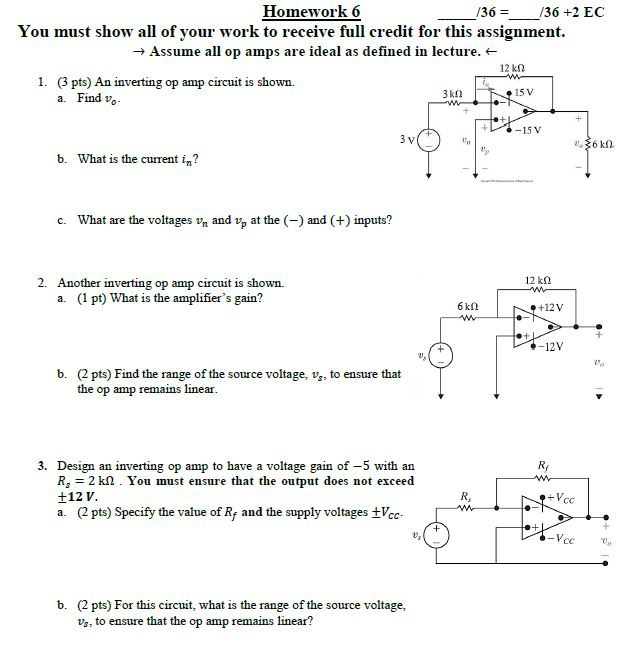# Question Homework 6 _/36 =__/36 +2 EC You must show all of your work to receive full credit for this assignment. → Assume all op amps are ideal as defined in lecture. + 1. (3 pts) An inverting op amp circuit is shown. a. Find vo- 39 3kN15V ***-15 "36k b. What is the current in? c. What are the voltages vm and v, at the (-) and (+) inputs? 12 k2 2. Another inverting op amp circuit is shown. a. (1 pt) What is the amplifier's gain? 6kA N +12V X-12V b. (2 pts) Find the range of the source voltage, vs, to ensure that the op amp remains linear. 3. Design an inverting op amp to have a voltage gain of -5 with an R = 2 k.2. You must ensure that the output does not exceed +12V. a. (2 pts) Specify the value of Rf and the supply voltages VCC N +Vcc Hot V -VC b. (2 pts) For this circuit, what is the range of the source voltage, Vs, to ensure that the op amp remains linear?TEEETI The Asker · Electrical EngineeringTranscribed Image Text: Homework 6 _/36 =__/36 +2 EC You must show all of your work to receive full credit for this assignment. → Assume all op amps are ideal as defined in lecture. + 1. (3 pts) An inverting op amp circuit is shown. a. Find vo- 39 3kN15V ***-15 "36k b. What is the current in? c. What are the voltages vm and v, at the (-) and (+) inputs? 12 k2 2. Another inverting op amp circuit is shown. a. (1 pt) What is the amplifier's gain? 6kA N +12V X-12V b. (2 pts) Find the range of the source voltage, vs, to ensure that the op amp remains linear. 3. Design an inverting op amp to have a voltage gain of -5 with an R = 2 k.2. You must ensure that the output does not exceed +12V. a. (2 pts) Specify the value of Rf and the supply voltages VCC N +Vcc Hot V -VC b. (2 pts) For this circuit, what is the range of the source voltage, Vs, to ensure that the op amp remains linear?
More
Transcribed Image Text: Homework 6 _/36 =__/36 +2 EC You must show all of your work to receive full credit for this assignment. → Assume all op amps are ideal as defined in lecture. + 1. (3 pts) An inverting op amp circuit is shown. a. Find vo- 39 3kN15V ***-15 "36k b. What is the current in? c. What are the voltages vm and v, at the (-) and (+) inputs? 12 k2 2. Another inverting op amp circuit is shown. a. (1 pt) What is the amplifier's gain? 6kA N +12V X-12V b. (2 pts) Find the range of the source voltage, vs, to ensure that the op amp remains linear. 3. Design an inverting op amp to have a voltage gain of -5 with an R = 2 k.2. You must ensure that the output does not exceed +12V. a. (2 pts) Specify the value of Rf and the supply voltages VCC N +Vcc Hot V -VC b. (2 pts) For this circuit, what is the range of the source voltage, Vs, to ensure that the op amp remains linear?